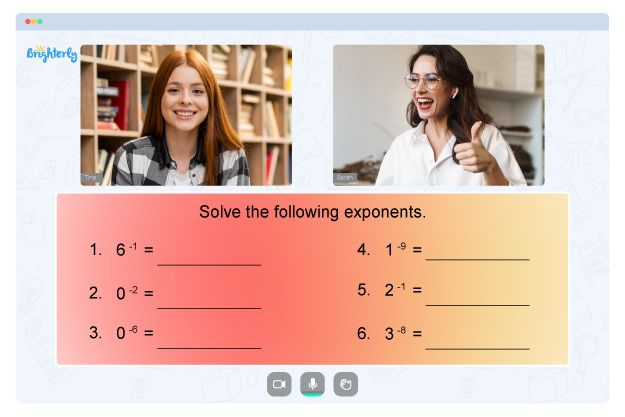# Round to the Nearest Hundredth

Welcome to Brighterly! We’re excited to guide you on a mathematical journey as we delve into the fascinating realm of decimals. Today’s lesson is all about learning how to round to the nearest hundredth. This crucial skill is invaluable when simplifying calculations, making estimations, and grasping the significance of numerical values. Join us as we embark on this adventure to become masters of rounding decimals!

## Rounding Decimals to the Nearest Hundredth

At Brighterly, we believe in making math engaging and fun. As we explore the process of rounding decimals, remember that we’re focusing on the nearest hundredth. The hundredth place is the second digit to the right of the decimal point and represents 1/100 (0.01) of a whole number. Rounding to the nearest hundredth entails scrutinizing the third decimal place (the thousandths place) and deciding whether to round up or down. By the end of this lesson, you’ll feel confident in your ability to round decimals and apply this skill in various mathematical contexts.

## Rounding Decimals to the Nearest Hundredth

Rounding decimals is the process of approximating a number to a specific decimal place. In this case, we’re focusing on the nearest hundredth. The hundredth place is the second digit to the right of the decimal point, representing 1/100 (0.01) of a whole number. Rounding to the nearest hundredth involves examining the third decimal place (the thousandths place) and determining whether to round up or down.

### How to Round to the Nearest Hundredth?

To round to the nearest hundredth, follow these simple steps:

1. Locate the hundredths place value (the second digit to the right of the decimal point).
2. Look at the digit in the thousandths place (the third digit to the right of the decimal point).
3. If the thousandths digit is 5 or greater, round up the hundredths digit by 1.
4. If the thousandths digit is less than 5, keep the hundredths digit unchanged.
5. Remove all the digits to the right of the hundredths place.

## Hundredths Place Value

Place value is the value of each digit in a number based on its position. In a decimal number, the hundredths place value is the second digit to the right of the decimal point, which represents 1/100 of a whole number. It’s essential to understand place values when working with decimals, as they help us read, write, and manipulate numbers more efficiently.

## Difference between Hundred and Hundredth

Though the words “hundred” and “hundredth” might sound similar, they represent entirely different concepts in mathematics. A hundred is a whole number equal to 100 units, whereas a hundredth refers to the decimal fraction 1/100, represented as 0.01. While a hundred is a significant number in our base-10 number system, a hundredth is a small fraction used to express parts of a whole.

## Round to the Nearest Hundredth Examples

Let’s go through some examples to solidify our understanding of rounding to the nearest hundredth:

1. Round 3.456 to the nearest hundredth:

Locate the hundredths digit (5) and look at the thousandths digit (6). Since 6 is greater than or equal to 5, round up the hundredths digit (5) by 1. The result is 3.46.

2. Round 12.034 to the nearest hundredth:

Locate the hundredths digit (3) and look at the thousandths digit (4). Since 4 is less than 5, keep the hundredths digit (3) unchanged. The result is 12.03.

## Practice Questions on Round to the Nearest Hundredth

It’s time to practice! Try rounding these decimals to the nearest hundredth:

1. 7.849
2. 2.015
3. 15.678
4. 0.952
5. 29.991

## Conclusion

Congratulations on completing this Brighterly lesson on rounding decimals to the nearest hundredth! With this essential skill under your belt, you’re now prepared to tackle more intricate math problems with ease. Rounding plays a vital role in simplifying calculations, making estimations, and deepening our comprehension of numerical values. As you continue to practice and apply your newfound knowledge, you’ll soon become a true rounding virtuoso. Remember, the Brighterly community is here to support you on your mathematical journey, so don’t hesitate to revisit our lessons and resources whenever you need a refresher. Happy learning!

## FAQs on Rounding to the Nearest Hundredth

### Why do we need to round decimals?

Rounding decimals is essential for simplifying calculations, estimating values, and understanding the significance of numbers. It allows us to work with approximate values, which are often more manageable and easier to interpret in real-world situations.

### Can you round to other decimal places besides the hundredths?

Absolutely! You can round to any decimal place, such as the nearest tenth, thousandth, or even beyond. The process is similar to rounding to the nearest hundredth; you just need to focus on the appropriate place value and the digit to the right of it.

### What’s the difference between rounding and truncating?

Rounding is the process of approximating a number to a specific decimal place based on the value of the next decimal place. Truncating, on the other hand, simply involves removing all the digits after a specific decimal place without considering their values. While rounding provides a more accurate approximation, truncating can lead to more significant errors in some cases.

Information Sources

For more information on rounding decimals, place values, and related concepts, you can explore the following resources:

Remember, practice makes perfect! Keep rounding decimals, and soon you’ll be a pro at approximating numbers to the nearest hundredth or any other decimal place. Happy learning with Brighterly!

Problems with Numbers?• Is your child finding it hard to master numbers fundamentals?
• An online tutor could be of help.

Does your child struggle with understanding the fundamentals of numbers? Try learning with an online tutor.